Courses

# Motion in One Dimension(Part- 2) - Physics, Solution by DC Pandey NEET Notes | EduRev

## NEET : Motion in One Dimension(Part- 2) - Physics, Solution by DC Pandey NEET Notes | EduRev

The document Motion in One Dimension(Part- 2) - Physics, Solution by DC Pandey NEET Notes | EduRev is a part of the NEET Course DC Pandey (Questions & Solutions) of Physics: NEET.
All you need of NEET at this link: NEET

Introductory Exercise 3.2

Ques 1: A ball is thrown vertically upwards. Which quantity remains constant among, speed, kinetic energy, velocity and acceleration?
Ans: acceleration
Sol: Acceleration (due to gravity).

Ques 2: Equation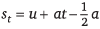does not seem dimensionally correct, why?
Ans: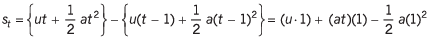Sol: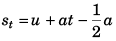is physically correct as itgives the displacement of the particle in tth second (or any time unit).
st = Displacement in t seconds - displacement in (t - 1) seconds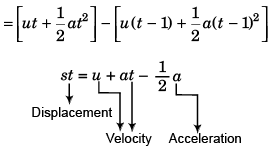Therefore, the given equation is dimensionally incorrect.

Ques 3: Can the speed of a particle increase as its acceleration decreases? If yes give an example.
Ans: Yes, in simple harmonic motion
Sol: Yes. When a particle executing simple harmonic motion returns from maximum amplitude position to its mean position the value of its acceleration decreases while speed increases.

Ques 4: The velocity of a particle moving in a straight line is directly proportional to 3/4th power of time elapsed. How does its displacement and acceleration depend on time?
Ans:  t7/4, t-1/4
Sol: v = t3/4 (given)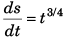…(i)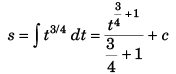or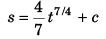i.e.,   s ∝ t7/4
Differentiating Eq. (i) w.r.t. time t,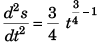⇒ a ∝ t-1/4

Ques 5: A particle is projected vertically upwards with an initial velocity of 40 m/s. Find the displacement and distance covered by the particle in 6 seconds. Take g = 10 m/s2.
Ans: 60 m, 100 m
Sol: Displacement (s) of the particle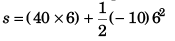= 240 - 180
= 60 m (in the upward direction)
Distance covered (D) by the particle
Time to attain maximum height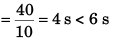It implies that particle has come back after attaining maximum height (h) given by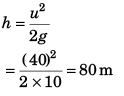∴ D = 80 + (80 - 60)
= 100 m

Ques 6: Velocity of a particle moving along positive x-direction is v = (40 - 101) m/s. Here, t is in seconds. A t time t = 0, the x coordinate of particle is zero. Find the time when the particle is at a distance of 60 m from origin.
Ans: 2 s, 6 s, 2 (2 + √7) s
Sol: v = 40 - 10t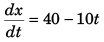or   dx = (40 - 10t) dt
or   x = ∫ (40 - 10t) dt
or    x = 40t - 5t2 + c
As at t = 0 the value of x is zero.
c = 0
∴ x = 40t - 5t2
For x to be 60 m.
60 = 40t - 5t2
or   t2 - 8t + 12 = 0
∴ t = 2 s or 6 s

Ques 7: A particle moves rectilinearly with initial velocity u and a constant acceleration a. Find the average velocity of the particle in a time interval from t = 0 to t = t second of its motion.
Ans: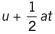Sol: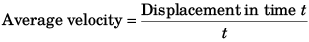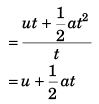Ques 8: A particle moves in a straight line with uniform acceleration. Its velocity at time t = 0 is v1 and at time t = t is v2. The average velocity of the particle in this time interval is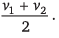Is this statement true or false?
Ans: True
Sol: v2 = v1 + at
∴ at = v2 - v1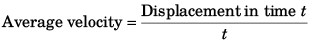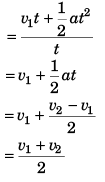Ques 9: Find the average velocity of a particle released from rest from a height of 125 m over a time interval till it strikes the ground, g = 10 m/s2.
Ans: 25 m/s (downwards)
Sol: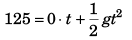⇒ t = 25 s
Average velocity = 125 m/5 s  (downwards)
= 25 m/s (downwards)

Ques 10: Velocity of a particle moving along x-axis varies with time as, v = (10 + 5t - t2) At time t = 0, x = 0.
Find
(a) acceleration of particle at t = 2 s
(b) x-coordinate of particle at t = 3 s
Ans: (a) 1 m/s2
(b) 43 .5 m
Sol: v = 10 + 5t - t2       …  (i)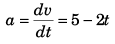At   t = 2 s
a = 5 - 2 x 2
= 1 m/s2
From Eq. (i),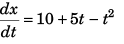∴ x = ∫ (10 + 5t - t2) dt
or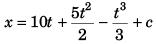As, at  t = 0 the value of x is zero
c = 0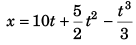Thus, at t = 3 s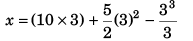= 30 + 22.5 - 9
= 43.5 m

Ques 11: Velocity of a particle at time t = 0 is 2 m/s. A constant acceleration of 2 m/s2 acts on the particle for 2 seconds at an angle of 60° with its initial velocity. Find the magnitude of velocity and displacement of particle at the end of t = 2 s.
Ans: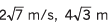Sol: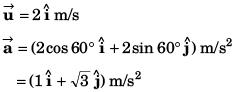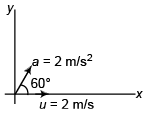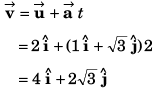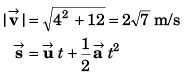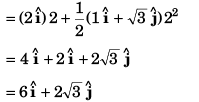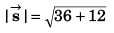= 4√3 m

Ques 12: Velocity of a particle at any time t is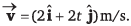Find acceleration and displacement of particle at t = 1 s. Can we apply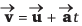or not?
Ans: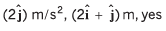Sol: Part I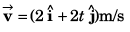…(i)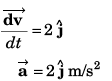From Eq. (i),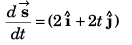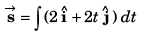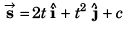Taking initial displacement to be zero.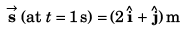Part II
Yes. As explained below.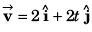implies that initial velocity of the particle is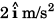and the acceleration is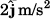∴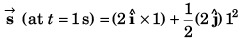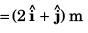Ques 13: The coordinates of a particle moving in x-y plane at any time t are (21, t2)..
Find:
(a) the trajectory of the particle,
(b) velocity of particle at time t and
(c) acceleration of particle at any time t.
Ans: (a) x2 = 4y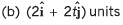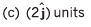Sol: x = 2t and y = t2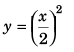or, x2 = 4y
(The above is the equation to trajectory) x = 2t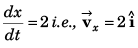y = t2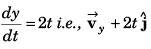Thus,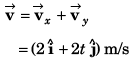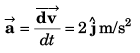Offer running on EduRev: Apply code STAYHOME200 to get INR 200 off on our premium plan EduRev Infinity!

210 docs

,

,

,

,

,

,

,

,

,

,

,

,

,

,

,

,

,

,

,

,

,

,

,

,

;